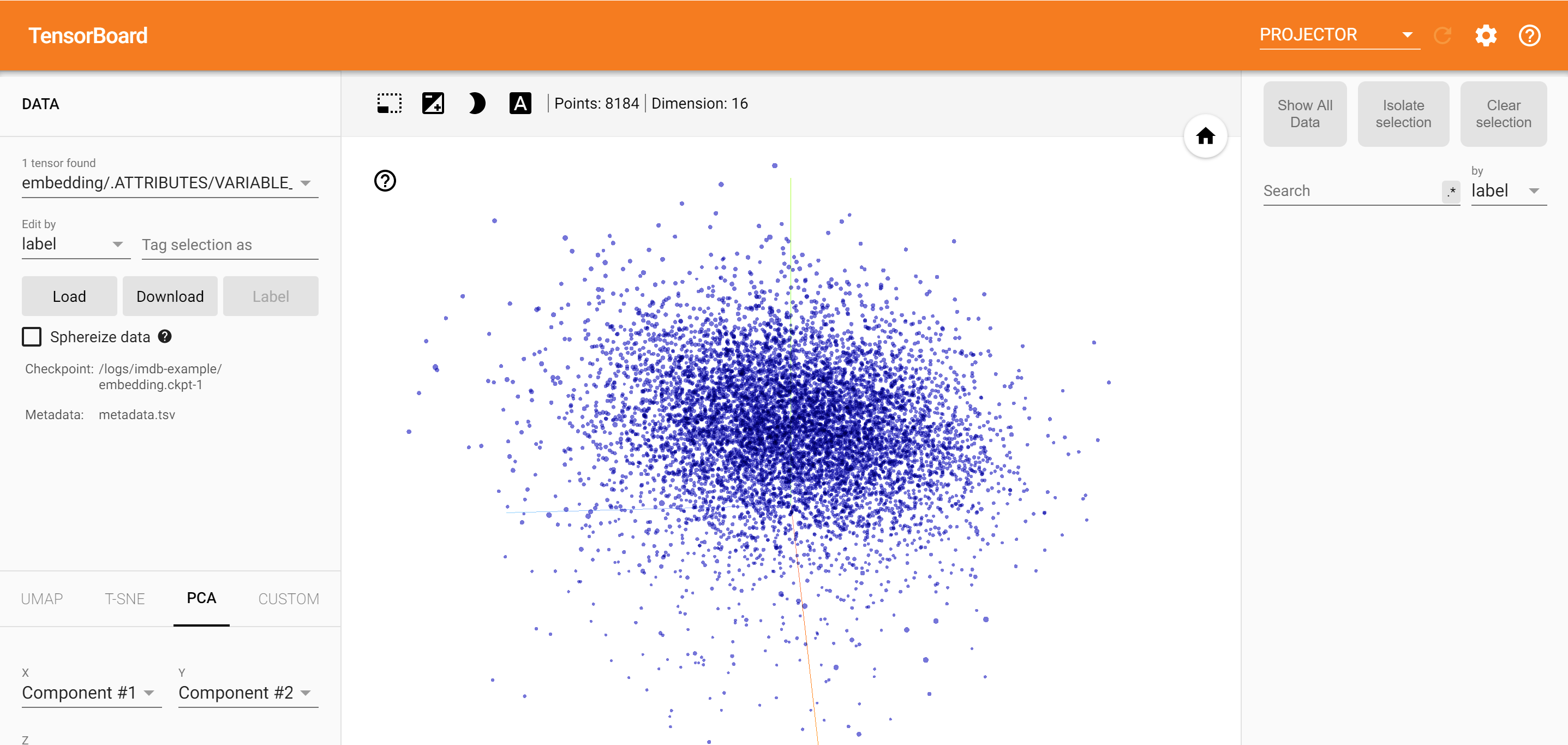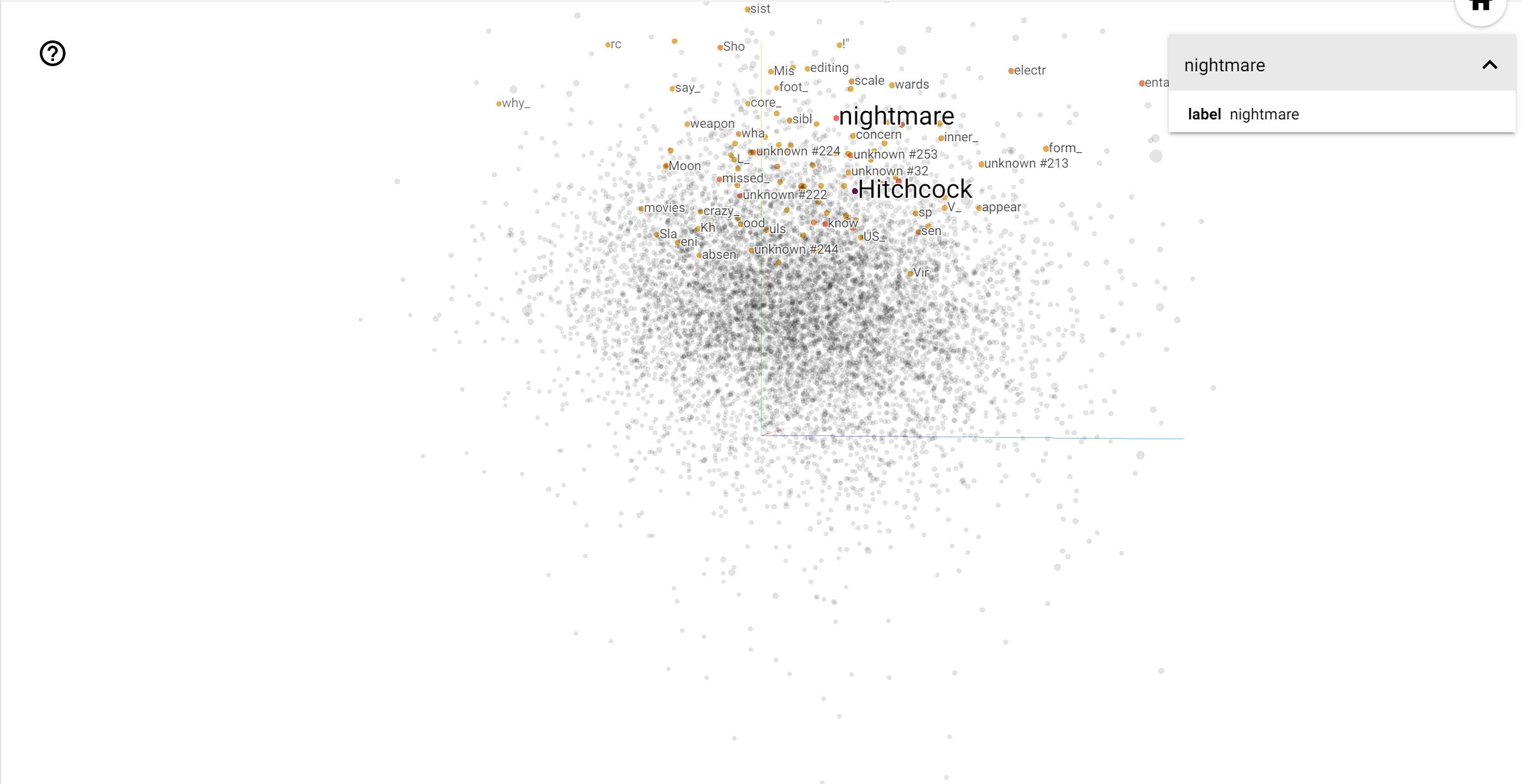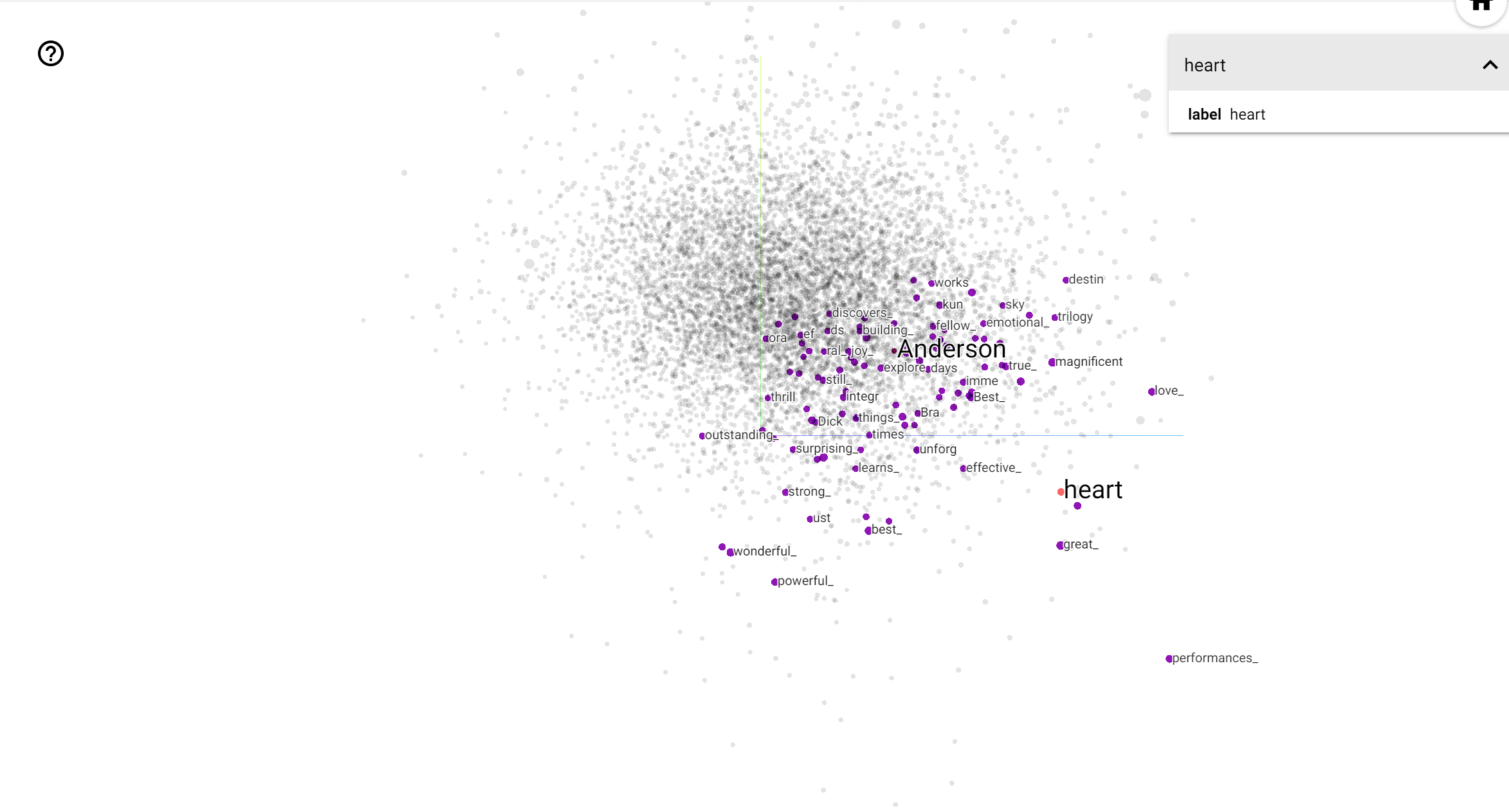# Visualizing Data using the Embedding Projector in TensorBoard

## Overview

Using the TensorBoard Embedding Projector, you can graphically represent high dimensional embeddings. This can be helpful in visualizing, examining, and understanding your embedding layers.In this tutorial, you will learn how visualize this type of trained layer.

## Setup

For this tutorial, we will be using TensorBoard to visualize an embedding layer generated for classifying movie review data.

``````try:
# %tensorflow_version only exists in Colab.
%tensorflow_version 2.x
except Exception:
pass

``````
``````import os
import tensorflow as tf
import tensorflow_datasets as tfds
from tensorboard.plugins import projector

``````

## IMDB Data

We will be using a dataset of 25,000 movies reviews from IMDB, labeled by sentiment (positive/negative). Reviews have been preprocessed, and each review is encoded as a sequence of word indexes (integers). For convenience, words are indexed by overall frequency in the dataset, so that for instance the integer "3" encodes the 3rd most frequent word in the data. This allows for quick filtering operations such as: "only consider the top 10,000 most common words, but eliminate the top 20 most common words".

As a convention, "0" does not stand for a specific word, but instead is used to encode any unknown word. Later in the tutorial, we will be removing this row from the visualization.

``````(train_data, test_data), info = tfds.load(
"imdb_reviews/subwords8k",
split=(tfds.Split.TRAIN, tfds.Split.TEST),
with_info=True,
as_supervised=True,
)
encoder = info.features["text"].encoder

# shuffle and pad the data.
)
)
train_batch, train_labels = next(iter(train_batches))

``````

# Keras Embedding Layer

A Keras Embedding Layer can be used to train an embedding for each word in your volcabulary. Each word (or sub-word in this case) will be associated with a 16-dimensional vector (or embedding) that will be trained by the model.

``````# Create an embedding layer
embedding_dim = 16
embedding = tf.keras.layers.Embedding(encoder.vocab_size, embedding_dim)
# Train this embedding as part of a keras model
model = tf.keras.Sequential(
[
embedding, # The embedding layer should be the first layer in a model.
tf.keras.layers.GlobalAveragePooling1D(),
tf.keras.layers.Dense(16, activation="relu"),
tf.keras.layers.Dense(1),
]
)

# Compile model
model.compile(
loss=tf.keras.losses.BinaryCrossentropy(from_logits=True),
metrics=["accuracy"],
)

# Train model
history = model.fit(
train_batches, epochs=1, validation_data=test_batches, validation_steps=20
)
``````
```2500/2500 [==============================] - 13s 5ms/step - loss: 0.5330 - accuracy: 0.6769 - val_loss: 0.4043 - val_accuracy: 0.7800

```

## Saving data for TensorBoard

TensorBoard reads tensors and metadata from your tensorflow projects from the logs in the specified `log_dir` directory. For this tutorial, we will be using `/logs/imdb-example/`.

In order to visualize this data, we will be saving a checkpoint to that directory, along with metadata to understand which layer to visualize.

``````# Set up a logs directory, so Tensorboard knows where to look for files
log_dir='/logs/imdb-example/'
if not os.path.exists(log_dir):
os.makedirs(log_dir)

# Save Labels separately on a line-by-line manner.
with open(os.path.join(log_dir, 'metadata.tsv'), "w") as f:
for subwords in encoder.subwords:
f.write("{}\n".format(subwords))
# Fill in the rest of the labels with "unknown"
for unknown in range(1, encoder.vocab_size - len(encoder.subwords)):
f.write("unknown #{}\n".format(unknown))

# Save the weights we want to analyse as a variable. Note that the first
# value represents any unknown word, which is not in the metadata, so
# we will remove that value.
weights = tf.Variable(model.layers.get_weights()[1:])
# Create a checkpoint from embedding, the filename and key are
# name of the tensor.
checkpoint = tf.train.Checkpoint(embedding=weights)
checkpoint.save(os.path.join(log_dir, "embedding.ckpt"))

# Set up config
config = projector.ProjectorConfig()
# The name of the tensor will be suffixed by `/.ATTRIBUTES/VARIABLE_VALUE`
embedding.tensor_name = "embedding/.ATTRIBUTES/VARIABLE_VALUE"
projector.visualize_embeddings(log_dir, config)
``````
``````%tensorboard --logdir /logs/imdb-example/
``````## Analysis

The TensorBoard Projector is a great tool for analyzing your data and seeing embedding values relative to each other. The dashboard allows searching for specific terms, and highlights words that are nearby in the embedding space. From this example we can see that Wes Anderson and Alfred Hitchcock are both rather neutral terms, but that they are referenced in different contexts.Hitchcock is closer associated to words like `nightmare`, which likely relates to his work in horror movies. While Anderson is closer to the word `heart`, reflecting his heartwarming style.